IMO Shortlist 2015 problem A6

Kvaliteta:
Avg: 0,0
Težina:
Avg: 8,0

Let$n$ be a fixed integer with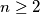$n \ge 2$. We say that two polynomials$P$ and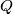$Q$ with real coefficients are block-similar if for each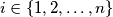$i \in \{1, 2, \ldots, n\}$ the sequences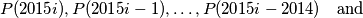$P(2015i), P(2015i - 1), \ldots, P(2015i - 2014) \quad \mbox{and}$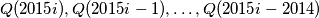$Q(2015i), Q(2015i - 1), \ldots, Q(2015i - 2014)$

are permutations of each other.

(a) Prove that there exist distinct block-similar polynomials of degree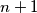$n + 1$.
(b) Prove that there do not exist distinct block-similar polynomials of degree$n$.